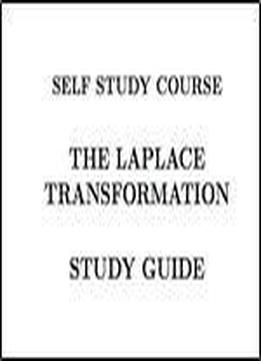# Laplace Transformation Self Study Course (dr. Alan Kraus Books For Engineers Book 1) by Alan Kraus / 2013 / English / EPUB

Students of Alan Kraus' The Laplace Transformation Self Study Course will be able to upon completion: Define Laplace transform Derive the Laplace transforms for the basic operations of the sums of transforms, the multiplication of a time function by a constant, differentiation and integration Derive the Laplace transforms for an exponentially damped function and a function shifted in time Summarize, and in part create, a tabulations of Laplace transform pairs Illustrate several methods for obtaining the inverse Laplace transform Show how to obtain the solutions to differential equations using the Laplace transform method Present additional useful concepts regarding the initial and final values of functions and the use for the Laplace transform variable as a differential and integral operator Show how to obtain solutions to differential equations which represent systems with more than one degree of freedom and for systems subjected to periodic forcing functions The course is 70 pages long. It also includes a 27 page self study guide and a 66 page Solutions to Skill Development Exercises.

views: 327